# Capacitance

## 6. Capacitors in series and parallel combinations

For prectical applications , two or more capacitors are often used in combination and their total capacitance C must be known.To find total capacitance of the arrangement of capacitor we would use equation
Q=CV
(i) Parallel combination of capacitors
• Figure below shows two capacitors connected in parallel between two points A and B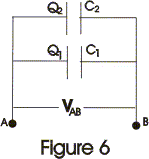• Right hand side plate of capacitors would be at same common potential VA. Similarly left hand side plates of capacitors would also be at same common potential VB.
• Thus in this case potential difference VAB=VA-VB would be same for both the capacitors, and charges Q1 and Q2 on both the capacitors are not necessarily equal. So,
Q1=C1V and Q2=C2V
• Thus charge stored is divided amongst both the capacitors in direct proportion to their capacitance.
• Total charge on both the capacitors is,
Q=Q1+Q2
=V(C1+C2)

and
Q/V=C1+C2                              (8)
So system is equivalent to a single capacitor of capacitance
C=Q/V
where,
• When capacitors are connected in parallel their resultant capacitance C is the sum of their individual capacitances.
• The value of equivalent capacitance of system is greater then the greatest individual one.
• If there are number of capacitors connected in parallel then their equivalent capacitance would be
C=C1+C2+ C3...........               (10)

(ii) Series combination of capacitors
• Figure 7 below shows two capacitors connected in series combination between points A and B.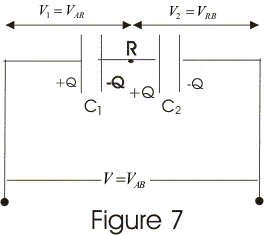• Both the points A and B are maintained at constant potential difference VAB.
• In series combination of capacitors right hand plate of first capacitor is connected to left hand plate of next capacitor and combination may be extended foe any number of capacitors.
• In series combination of capacitors all the capacitors would have same charge.
• Now potential difference across individual capacitors are given by
VAR=Q/C1
and,
VRB=Q/C2
• Sum of VAR and VRB would be equal to applied potential difference V so,
V=VAB=VAR+VRB
=Q(1/C1 + 1/C2)

or,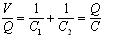where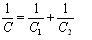i.e., resultant capacitance of series combination C=Q/V, is the ratio of charge to total potential difference across the two capacitors connected in series.
• So, from equation 12 we say that to find resultant capacitance of capacitors connected in series, we need to add reciprocals of their individual capacitances and C is always less then the smallest individual capacitance.
• Result in equation 12 can be summarized for any number of capacitors i.e.,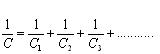## 7. Energy stored in a capacitor

• Consider a capacitor of capacitance C, completely uncharged in the begning.
• Charhing process of capacitor requires expanditure of energy because while charging a capacitor charge is transferred from plate at lower potential to plate at higher potential.
• Now if we start charging capacitor by transporting a charge dQ from negative plate ti the positive plate then work is done against the potential difference across the plate.
• If q is the amount of charge on the capacitor at any stage of charging process and φ is the potential difference across the plates of capacitor then magnitude of potential difference is φ=q/C.
• Now work dW required to transfer dq is
dW=φdq=qdq/C
• To charge the capacitor starting from the uncharged state to some final charge Q work required is
Integrating from 0 to Q
W=(1/C)∫qdq
=(Q2)/2C
(14a)
=(CV2)/2
=QV/2

Which is the energy stored in the capacitor and can also be written as
U=(CV2)/2 ---(15)

• From equation 14c,we see that the total work done is equal to the average potential V/2 during the charging process ,multiplied by the total charge transferred
• If C is measured in Farads ,Q in coulumbs and V in volts the energy stored would in Joules
• A parallel plate capacitor of area A and seperation d has capacitance

C=ε0A/d

• electric field in the space between the plates is
E=V/d or V=Ed

Putting above values of V and C in equation 14b we find
W=U=(1/2)(ε0A/d)(Ed)2
=(1/2)ε0E2.V
---(16)

• If u denotes the energy per unit volume or energy density then
u=(1/2)ε0E2 x volume
• The result for above equation is generally valid even for electrostatic field that is not constant in space.